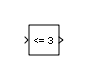# Compare To Constant

Determine how signal compares to specified constant

•Libraries:
Simulink / Logic and Bit Operations
HDL Coder / Logic and Bit Operations

## Description

The Compare To Constant block compares an input signal to a constant. Specify the constant in the Constant value parameter. Specify how the input is compared to the constant value with the Operator parameter.

## Ports

### Input

expand all

Input signal, specified as a scalar, vector, or matrix, is compared with constant. The block first converts its Constant value parameter to the input data type, and then performs the specified operation.

Data Types: `half` | `single` | `double` | `int8` | `int16` | `int32` | `int64` | `uint8` | `uint16` | `uint32` | `uint64` | `Boolean` | `fixed point` | `enumerated` | `bus`

### Output

expand all

The output is `0` if the comparison is false, and `1` if it is true.

Data Types: `uint8` | `Boolean`

## Parameters

expand all

This parameter can have these values:

• `==` — Determine whether the input is equal to the specified constant.

• ~`=` — Determine whether the input is not equal to the specified constant.

• `<` — Determine whether the input is less than the specified constant.

• `<=` — Determine whether the input is less than or equal to the specified constant.

• `>` — Determine whether the input is greater than the specified constant.

• `>=` — Determine whether the input is greater than or equal to the specified constant.

#### Programmatic Use

 Block Parameter: `relop` Type: character vector Values: `'=='` | `'~='`| `'<'` |`'<='`| `'>='`| `'>'` Default: `'<='`

Specify the constant value to which the input is compared.

#### Programmatic Use

 Block Parameter: `const` Type: character vector Value: scalar | vector | matrix | N-D array Default: `'3.0'`

Specify the data type of the output, `boolean` or `uint8`.

#### Programmatic Use

 Block Parameter: `OutDataTypeStr` Type: character vector Values:`'boolean'` | `'uint8'` Default: `'boolean'`

#### Programmatic Use

 Block Parameter: `ZeroCross` Type: character vector Values: `'off'` | `'on'` Default: `'on'`

## Block Characteristics

 Data Types `Boolean` | `double` | `enumerated` | `fixed point` | `half` | `integer` | `single` Direct Feedthrough `yes` Multidimensional Signals `yes` Variable-Size Signals `yes` Zero-Crossing Detection `yes`

## Version History

Introduced before R2006a